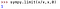# Fixing SymPy’s Limit Calculator Flaw

SymPy is a Python library written entirely in python that aims to become a full-featured computer algebra system (CAS) while keeping it’s code as simple as possible.

If you ever have to do advanced math things with Python i’d highly recommend SymPy. It's capable of solving equations, plotting graphs, taking integrals and most other math’y things you can think of.

A limit is the value that a function “approaches” as the input(x value) “approaches” some value. For example, the limit of x/x as x approaches zero would be written as follows

The format of SymPy’s limit function is as follows

For this particular limit our function call would look as follows:We did not include the optional direction parameter because our limit approaches from both directions(sympys default)

Our function graphed looks as follows:

Although our function is not defined at x=0 because dividing by zero is indeterminate. It does appear that our function approaches 1 as x approaches zero, so SymPy does appear correct in this instance.

# The Problem

If we take a function that has a limited domain such as the square root of x and we try to find the limit as our function approaches zero from the left, SymPy gives us the following result:

But if we look at the graph i’ve attached below, sqrt(x) has a domain from [0,+∞) so it is literally impossible to approach from the left.

SymPy doesn't respect the functions domain when calculating limits

Another case where SymPy will give us incorrect results is if we take a function like x/|x| which i’ve graphed below and try to take its limit as x approaches zero.

This result is obviously incorrect as our function approaches a different value from the left than it does from the right. If we take the one-sided limits with SymPy we get:

These limits are correct, since the two one sided limits are different, the two-sided limit does not exist.

SymPy struggles to take limits of any function containing jump discontinuities

# Explanation

This occurs because of the way SymPy calculates limits. SymPy uses series expansion to approximate the functions value at the desired point. Here’s a video by PatrickJMT solving a limit using series expansion. This is inherently flawed if the function isn't “well-behaved” in the neighborhood around our point. Since series expansion is just an approximation, the location of any jump discontinuity(s) maybe shifted every so slightly in either direction. This method also fails to take into account any domain issues our limit may face.

# The Solution

Our solution will be to approximate the limit by calculating a point on the appropriate side(s) of our desired limit that is incredibly close to the value we are approaching to see if the limit exists.

We will start off by differentiating our 3 cases, left limit, right limit, normal(bi-directional) limit.

If it’s a limit from the left we are going to test if our function is defined at a number slightly smaller than the one we are approaching, I will do 1e-8 to the left of our number.(1e-8 is incredibly small, it represents 1/one hundred million). To verify that our function is defined to the left of our desired point we want to check if that number is real, if so, we will run the SymPy function if not we will return an error message.

Based on our knowledge of limits the first one should be an error and the second should be a number close to zero

Seems as if we are doing ok so far. Now we will expand this concept to right sided limits by adding our very small number(1e-8) to the number we are approaching to obtain a point thats to the right of our limit.

Now for our final situation the two-sided limit, we will calculate the absolute difference between the two one sided limits, if its small enough we will assume that the limit exists and run SymPy, otherwise we will return an error.

Note how we don't include approachFrom in our else statement as a parameter for sympy.limit because the two sided limit is the default one.

We can now test our code our with some of the problems we found above:

I would like to take a moment to point out that our function is conceptually very similar to the epsilon delta definition of a limit. Which to summarize states that if the limit exists, the closer we get to our point from either side(horizontally), the smaller the range of our function on that interval becomes. So as our new domain becomes smaller (centered around the x value we are approaching) the range of our function using that domain will eventually become so small that it will approach a single number which is what we call our limit.

## The Startup